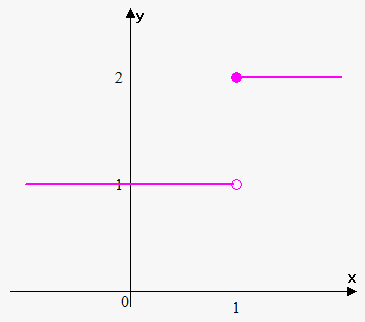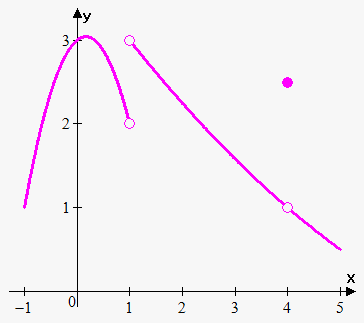# One-Sided Limits

## Related Calculator: Limit Calculator

Now we can extend concept of limit.

Definition. We write that lim_(x->a^-)f(x)=L and say "the limit of f(x), as x approaches a from the left, equals L" if for any epsilon>0 there exists delta>0 such that |f(x)-L|<epsilon when |x-a|<delta and x>a.

In other words we are interested in behavior of function near a and only to the left from a.

Definition. We write that lim_(x->a^+)f(x)=L and say "the limit of f(x), as x approaches a from the right, equals L" if for any epsilon>0 there exists delta>0 such that |f(x)-L|<epsilon when |x-a|<delta and x<a.

In other words we are interested in behavior of function near a and only to the right from a.

One-sided limits often arise for piecewise defined functions.

Example 1. Find lim_(x->1^+)f(x), lim_(x->1^-)f(x), lim_(x->1)f(x) where f(x)={(2 if x>=1),(1 if x<1):}.As x approaches 1 from the left f(x) approaches 1, thus lim_(x->1^-)f(x)=1.

As x approaches 1 from the right f(x) approaches 2, thus lim_(x->1^+)f(x)=2.

So, there is no single number that f(x) approaches as x approaches 1, thus lim_(x->1)f(x) doesn't exist.

Fact. lim_(x->a)f(x)=L if and only if lim_(x->a^-)f(x)=lim_(x->a^+)f(x)=L.

This fact is quite useful because often it is easier to find one-sided limits and to check whether they are equal to make conclusion about limit.

Example 2. The graph of the function f(x) is shown. Find (if they exist) values of the following limits:1. lim_(x->1^-)f(x)
2. lim_(x->1^+)f(x)
3. lim_(x->1)f(x)
4. lim_(x->4^-)f(x)
5. lim_(x->4+)f(x)
6. lim_(x->4)f(x)

From graph it is seen that f(x) approaches 2 as x approaches 1 from the left and f(x) approaches 3 when x approaches 1 from the right.

Therefore, lim_(x->1^-)f(x)=2 and lim_(x->1^+)f(x)=3.

Since lim_(x->1^-)f(x)!=lim_(x->1^+)f(x) then lim_(x->1)f(x) doesn't exist.

Also from graph it can be seen that f(x) approaches 1 as x approaches 4 from the left and f(x) approaches 1 when x approaches 4 from the right. Therefore, lim_(x->4^-)f(x)=1 and lim_(x->4^+)f(x)=1.

Since lim_(x->4^-)f(x)=lim_(x->4^+)f(x)=1 then lim_(x->4)f(x)=1.

Despite this fact notice that f(4)!=1.

Example 3. Find lim_(x->0)|x|.

Recall that |x|={(x if x>=0),(-x if x<0):}.

Therefore, for x>0 |x|=x, thus lim_(x->0+)|x|=lim_(x->0^+)x=0.

Since for x<0 |x|=-x, then lim_(x->0^-)|x|=lim_(x->0^-)-x=0.

We see that lim_(x->0^+)|x|=lim_(x->0^-)|x|=0. That's why lim_(x->0)|x|=0.

Example 4. Find lim_(x->0)(|x|)/x.

For x>0 |x|=x, thus lim_(x->0+)(|x|)/x=lim_(x->0^+)x/x=lim_(x->0^+)1=1.

For x<0 |x|=-x, thus lim_(x->0^-)(|x|)/x=lim_(x->0^-)(-x)/x=lim_(x->0^-)-1=-1.

We see that lim_(x->0^+)(|x|)/x!=lim_(x->0^-)(|x|)/x. That's why lim_(x->0)(|x|)/x doesn't exist.